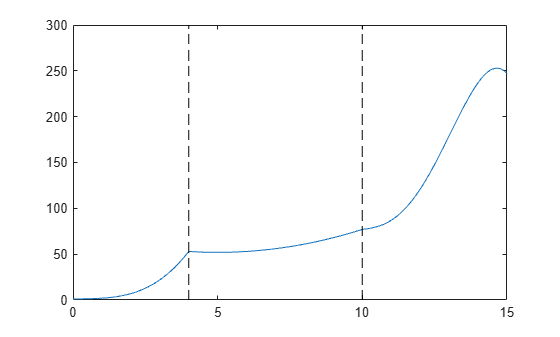ppval

Evaluate piecewise polynomial

Description

example

v = ppval(pp,xq) evaluates the piecewise polynomial pp at the query points xq.

Examples

collapse all

Create a piecewise polynomial that has a cubic polynomial in the interval [0,4], a quadratic polynomial in the interval [4,10], and a quartic polynomial in the interval [10,15].

breaks = [0 4 10 15];
coefs = [0 1 -1 1 1; 0 0 1 -2 53; -1 6 1 4 77];
pp = mkpp(breaks,coefs)
pp = struct with fields:
form: 'pp'
breaks: [0 4 10 15]
coefs: [3x5 double]
pieces: 3
order: 5
dim: 1

Evaluate the piecewise polynomial at many points in the interval [0,15] and plot the results. Plot vertical dashed lines at the break points where the polynomials meet.

xq = 0:0.01:15;
plot(xq,ppval(pp,xq))
line([4 4],ylim,'LineStyle','--','Color','k')
line([10 10],ylim,'LineStyle','--','Color','k')Create and plot a piecewise polynomial with four intervals that alternate between two quadratic polynomials.

The first two subplots show a quadratic polynomial and its negation shifted to the intervals [-8,-4] and [-4,0]. The polynomial is

$1-{\left(\frac{x}{2}-1\right)}^{2}=\frac{-{x}^{2}}{4}+x.$

The third subplot shows a piecewise polynomial constructed by alternating these two quadratic pieces over four intervals. Vertical lines are added to show the points where the polynomials meet.

subplot(2,2,1)
cc = [-1/4 1 0];
pp1 = mkpp([-8 -4],cc);
xx1 = -8:0.1:-4;
plot(xx1,ppval(pp1,xx1),'k-')

subplot(2,2,2)
pp2 = mkpp([-4 0],-cc);
xx2 = -4:0.1:0;
plot(xx2,ppval(pp2,xx2),'k-')

subplot(2,1,2)
pp = mkpp([-8 -4 0 4 8],[cc;-cc;cc;-cc]);
xx = -8:0.1:8;
plot(xx,ppval(pp,xx),'k-')
hold on
line([-4 -4],ylim,'LineStyle','--')
line([0 0],ylim,'LineStyle','--')
line([4 4],ylim,'LineStyle','--')
hold offInput Arguments

collapse all

Piecewise polynomial, specified as a structure. You can create pp using spline, pchip, makima, interp1, or the spline utility function mkpp.

Query points, specified as a vector or array. xq specifies the points where ppval evaluates the piecewise polynomial.

Data Types: single | double

Output Arguments

collapse all

Piecewise polynomial values at query points, returned as a vector, matrix, or array.

If pp has [d1,..,dr]-valued coefficients (nonscalar coefficient values), then:

• When xq is a vector of length N, v has size [d1,...,dr,N], and v(:,...,:,j) is the value at xq(j).

• When xq has size [N1,...,Ns], v has size [d1,...,dr,N1,...,Ns], and v(:,...,:, j1,...,js) is the value at xq(j1,...,js).